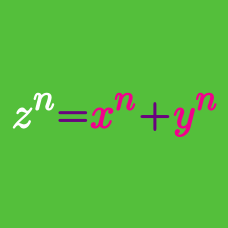Number Theory

# General Diophantine Equations: Level 5 Challenges

An $x \times y \times z$ rectangular prism is made out of $xyz$ identical unit cubes. Divide these cubes into 2 mutually exclusive sets.

The first set contains all cubes which have at least one face that is a constituent of the exterior faces of the rectangular prism. These cubes will be called external.

The second set contains all cubes which are completely enclosed inside the rectangular prism. These cubes will be called internal.

An example is that in a $3 \times 4 \times 7$ rectangular prism, there are 74 external cubes and 10 internal cubes.

How many different rectangular prisms are there, whose number of internal cubes is equal to the number of external cubes?

If you think the answer is infinite, type -1.

Details and Assumptions:

• Rotations/Flips among any axis are considered the same.

• Rectangular prisms can have square faces

$y^2(x^2+y^2-2xy-x-y) = (x+y)^2 (x-y)$

Find the number of integer pairs $(x, y)$ that satisfy the above expression.

$\large (p-4)(p+1)(p+3)=(n-4)(n+4)$

Find the sum of all primes $p$ satisfying the equation above, with $n$ is a positive integer.

A positive integer $n$ is funny if for each of its positive divisors $d$, the number $d+2$ is prime. Find the sum of all funny numbers that have the most quantity of divisors.

$\large x^2(y^3+z^3)=315(xyz+7)$Let $(x_1, y_1, z_1), (x_2, y_2, z_2), \cdots , (x_n, y_n, z_n)$ be all the solutions to the given equation, where $x>3$, $y$ and $z$ are positive integers. Find$\sum_{k=1}^{n} (x_k+y_k+z_k) \; .$

×

Problem Loading...

Note Loading...

Set Loading...